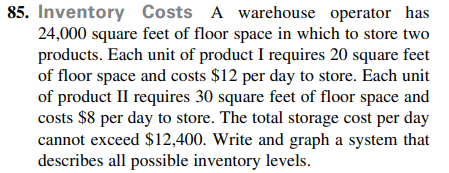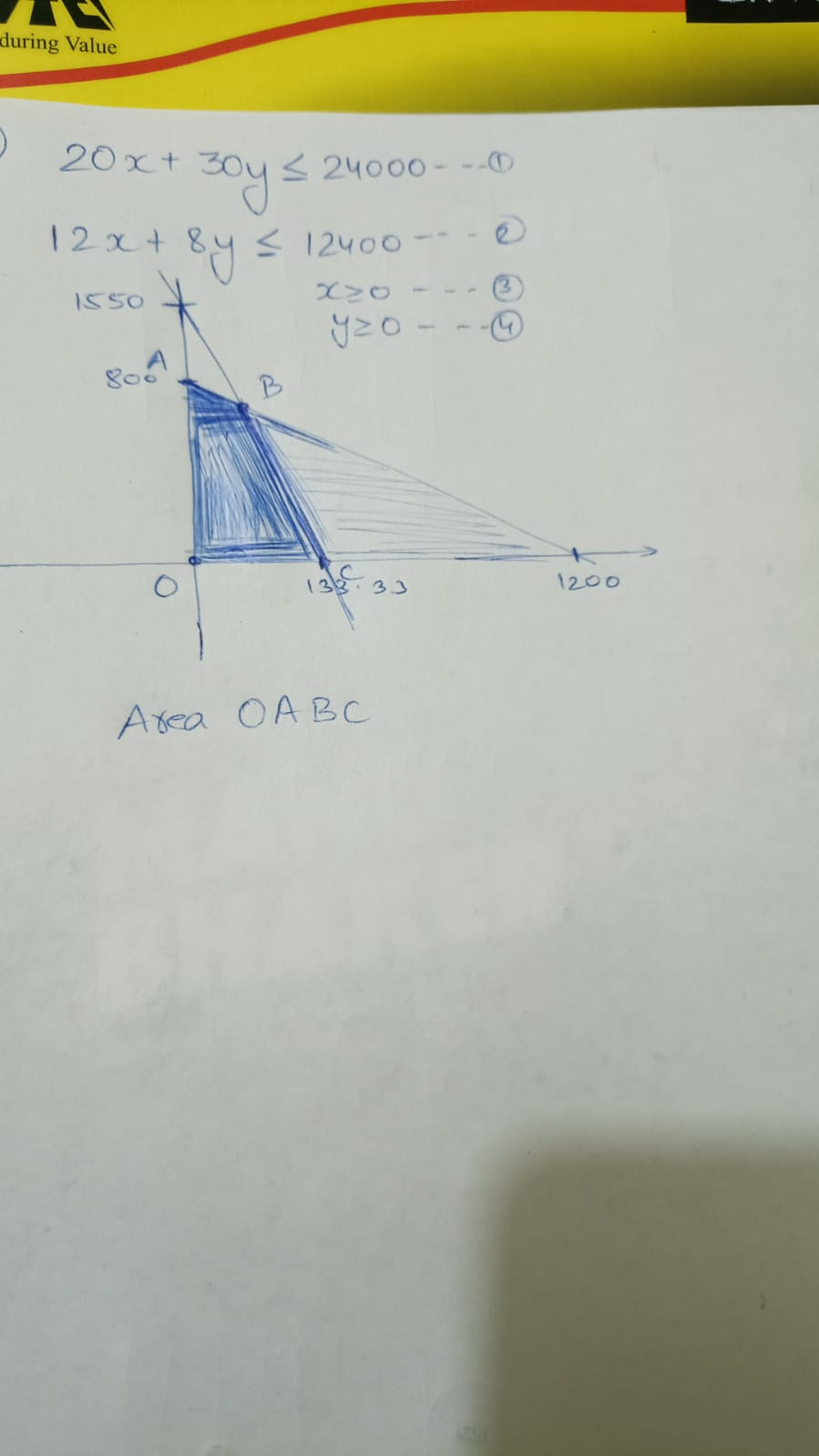### Still have math questions?

Algebra
QuestionInventory Costs A warehouse operator has

$$24,000$$ square feet of floor space in which to store two products. Each unit of product I requires $$20$$ square feet of floor space and costs $$\ 12$$ per day to store. Each unit of product II requires $$30$$ square feet of floor space and costs $$\ 8$$ per day to store. The total storage cost per day cannot exceed $$\ 12,400$$ . Write and graph a system that describes all possible inventory levels.